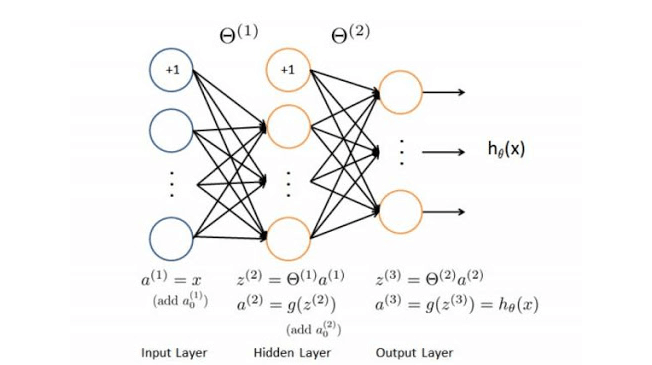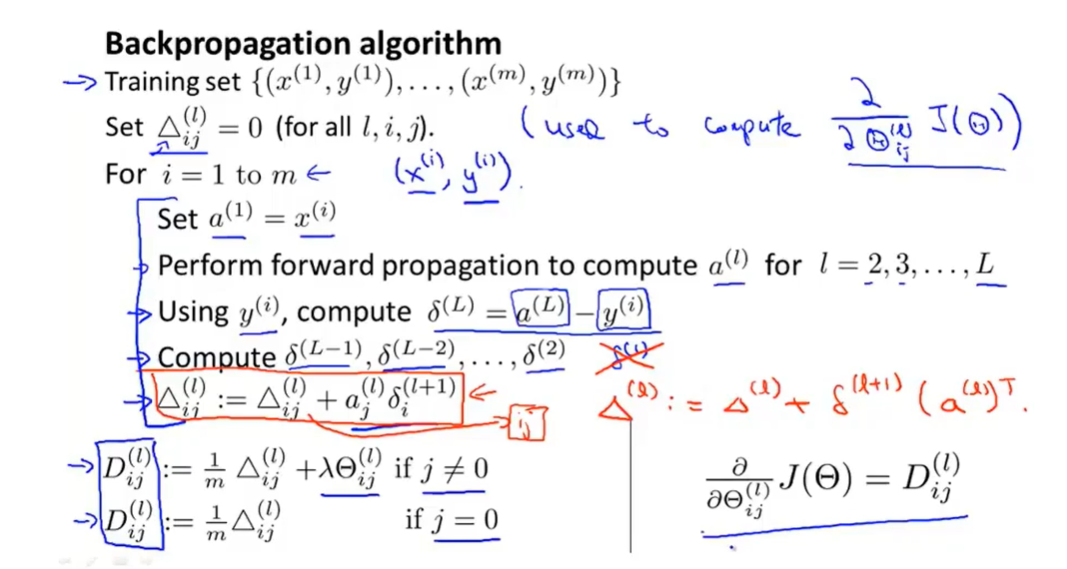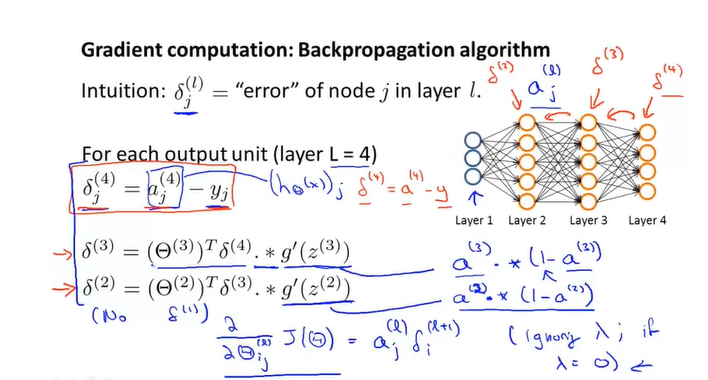星期五, 一月 31日 2020, 8:06 晚上

4.4k 字     19 分钟

# 反向传播算法

——来自Wiki百科的定义

## 算法流程详解

-注：此处以吴恩达机器学习视频中讲解的算法首先进行阐述。• $x_j$仅仅表示最左侧输入层的输入，下标$j$表示第$j$个单元(unit)。

• $\theta_{i,j}^{(l)}$表示从第$(l)$层的第$i$个单元到第$(l+1)$层的第j个单元的$\theta$

• $\theta^{(l)}$$\theta_{i,j}^{(l)}$的矩阵形式。$\theta^{(l)}$中的每一行（$i$相同）是$a_i^{(l)}$所散发给下一层的参数，是$a_j^{(l)}$的接收参数

• $l$表示网络的总层数，$l=$网络总层数

• $a_{j}^{(l)}$表示表示第$l$层的第$j$个神经元的输出

• $z_{j}^{(l)}$表示表示第$l$层的第$j$个神经元的输入

• $a_{j}^{(l)}=g^{\prime}\left(z_{j}^{(l)}\right)=\operatorname{sigmoid}^{\prime}\left(z_{j}^{(l)}\right)$其中sigmoid是激活函数

• $a_{j}^{(l-1)}$$z_{j}^{(l)}$的转化如图所示

• $C(\Theta)$是代价函数，在这里

$J(\Theta)=C(\Theta)=\\-\frac{1}{m} \sum_{i=1}^{m} \sum_{k=1}^{k}\left[y_{k}^{(i)} \log \left(\left(h_{\Theta}\left(x^{(i)}\right)\right)_{k}\right)+\left(1-y_{k}^{(i)}\right) \log \left(1-\left(h_{\Theta}\left(x^{(i)}\right)\right)_{k}\right)\right]\\+\frac{\lambda}{2 m} \sum_{l=1}^{L-1} \sum_{i=1}^{s l} \sum_{j=1}^{s_{(l+1)}}\left(\Theta_{j, i}^{(l)}\right)^{2}$

• $s_{(l)}$表示$a^{(l)}$神经元的个数

• 当然你也可以使用其他的代价函数，还有一个常用的代价函数是二次代价函数：

$C=\frac{1}{2 n} \sum_{x}\left\|y(x)-a^{L}(x)\right\|^{2}$1. 首先第一行没什么说的，就是目前有m个训练样本，接下来我们要对这m个训练样本进行一些神奇的操作，使得构建的神经网络更加准确。

2. 第二行设置了一个变量，每一个$\triangle_{i j}^{(l)}$都对应着$\theta_{i, j}^{(l)}$，这里$\triangle_{i j}^{(l)}$只是用来计算$\frac{\partial}{\partial \Theta_{i j}^{(l)}} J(\Theta)$的一个变量，后面我会详细进行解释。

3. 第三行是一个循环语句，对于每一对训练数据$\left(x^{(i)}, y^{(i)}\right)$(一共m个),都进行如下的操作。

4. 首先将训练数据的x输入，也就是$a^{(1)}=x^{(i)}$

5. 首先进行正向传播（假设$\theta_{i,j}^{(l)}$都有初值，这里的初值后面还会通过反向传播进行更新，应该是可以人为随意确定），这里过程就忽略了，总之通过正向传播我可得到每一层的$a^{(l)}$$z_{j}^{(l)}$（当$l=2,3, \ldots, L$的时候）

6. 下面开始反向传播的过程，首先计算一个值$\delta^{(L)}$，我们通常把这个值叫做"误差"，每一个神经元以及每一对训练数据都会对应一个误差值，关于这个误差值为什么这么计算，以及如何用最后一层的误差值来计算前面几层的误差值（其实就是所谓的反向传播），我会放在后文来解释。总之这个目前我们可以理解为，$\delta$用来表示与神经元计算结果与正确结果的偏差值，由于计算结果（最后一层）存在"误差"，所以将最后一层的"误差"通过一定的计算方法来反推各个神经元的"误差"，然后利用每个神经元的每个训练样本所找到的误差之和来更新$\theta_{i, j}^{(l)}$，这就是我们反向传播的基本目标，后面我会详细讲解这之中的公式。最后一层"误差"的计算公式是这样的：$\delta^{(L)}=a^{(L)}-y$
其中L表示最后一层（输出层），这个公式看起来比较合理，你可能会想为什么要这么计算，请 不要着急，关于为什么这么计算，它是有一定的道理的，我会放在后面进行解释。

7. 下面将进行与正向传播相似的过程，计算$\delta^{(L-1)}, \delta^{(L-2)}, \ldots, \delta^{(2)}$，(计算这些参数的算法我将在后文进行具体的阐述)，当然第一层就是输入层，也不存在所谓的"误差"，所以并不需要考虑$\delta^{(1)}$
然后将每个训练样本计算出来的误差先乘以$a_{j}^{(l)}$再进行累加，这个大概的意思是将每个训练样本存在的误差都综合起来，后文我会详细阐述为什么要这么做。

8. 我们进行最后一步操作（关于为什么要这么做，我会在后面进行详细的解释。）：

\begin{aligned} &\cdot D_{i, j}^{(l)}:=\frac{1}{m}\left(\Delta_{i, j}^{(l)}+\lambda \Theta_{i, j}^{(l)}\right), i f j \neq 0\\ &\cdot D_{i, j}^{(l)}:=\frac{1}{m} \Delta_{i, j}^{(l)}, i f j=0 \end{aligned}

$\frac{\partial}{\partial \Theta_{i j}^{(l)}} J(\Theta)=D_{i j}^{(l)}$

## 关于$\delta$

$\delta$在很多书中翻译为‘误差’，但这与模型预测值与样本y之间的误差不是一个概念。实际上$\delta$是一个微分值。书上将其定义为：

$\delta(l)=\frac{\partial C}{\partial z^{(l)}}$

$\frac{\partial C}{\partial \theta^{(l)}}=\frac{\partial C}{\partial z^{(l+1)}} \cdot \frac{\partial z^{(l+1)}}{\partial \theta^{(l)}}=\delta^{(l+1)} \cdot \frac{\partial z^{(l+1)}}{\partial \theta^{(l)}}$

$\frac{\partial z^{(l+1)}}{\partial \theta^{(l)}}$恰好等于$a^{(l)}$（线性函数求导）。

$\frac{\partial C}{\partial \theta^{(l)}}=\delta^{(l+1)} \cdot a^{(l)}$

## 寻找层与层之间"误差"的关系

$z^{(l+1)}=\theta^{(l)} \cdot \text { sigmoid }\left(z^{(l)}\right)$

$\text {sigmoid}^{\prime}\left(z^{(l)}\right)=a^{(l)} \cdot\left(1-a^{(l)}\right)$

$\delta^{(l)}=\frac{\partial C}{\partial z^{(l)}}=\frac{\partial C}{\partial z^{(l+1)}} \cdot \frac{\partial z^{(l+1)}}{\partial z^{(l)}}=\\\delta^{(l+1)} \cdot\left(\theta^{(l)}\right)^{T} \cdot a^{(l)} \cdot\left(1-a^{(l)}\right)$## 确定输出层的"误差"

$\delta(l)=\frac{\partial C}{\partial z^{(l)}}$

$J(\Theta)=C(\Theta)=\\-\frac{1}{m} \sum_{i=1}^{m} \sum_{k=1}^{k}\left[y_{k}^{(i)} \log \left(\left(h_{\Theta}\left(x^{(i)}\right)\right)_{k}\right)+\left(1-y_{k}^{(i)}\right) \log \left(1-\left(h_{\Theta}\left(x^{(i)}\right)\right)_{k}\right)\right]\\+\frac{\lambda}{2 m} \sum_{l=1}^{L-1} \sum_{i=1}^{s l} \sum_{j=1}^{s_{(l+1)}}\left(\Theta_{j, i}^{(l)}\right)^{2}$

$J(\theta)=C(\theta)=-\left[y \cdot \log \left(a^{(L)}\right)+(1-y) \log \left(1-a^{(L)}\right)\right]$

$\frac{\partial C}{\partial z^{(L)}}=\frac{\partial J(\Theta)}{\partial a^{(L)}} \cdot \frac{\partial a^{(L)}}{\partial z^{(L)}}$

$\frac{\partial C}{\partial a^{(L)}}=\frac{a^{(L)}-y}{\left(1-a^{(L)}\right) \cdot a^{(L)}}$

$\frac{\partial a^{(L)}}{\partial z^{(L)}}=a^{(L)} \cdot\left(1-a^{(L)}\right)$

$\frac{\partial C}{\partial z^{(L)}}=\delta^{(L)}=a^{(L)}-y$

## 笔者补充

$\frac{\partial C}{\partial \theta^{(l)}}=\delta^{(l+1)} \cdot a^{(l)}$

\begin{aligned} &\cdot D_{i, j}^{(l)}:=\frac{1}{m}\left(\Delta_{i, j}^{(l)}+\lambda \Theta_{i, j}^{(l)}\right), i f j \neq 0\\ &\cdot D_{i, j}^{(l)}:=\frac{1}{m} \Delta_{i, j}^{(l)}, i f j=0 \end{aligned}

## 参考"觉得赞的话，微信扫一扫赞赏一下吧"

Plugins